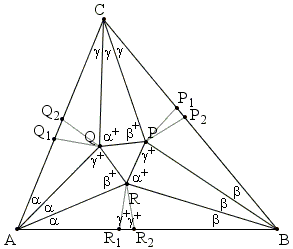Morley's MiracleB. Bollobas' proof

This proof appears in The Art of Mathematics by B. Bollobás (Cambridge University Press, 2006, p. 127-128) accompanied by a remark that the author was used to offer Morley's theorem in the early 1970s as a problem to his better freshmen in Cambridge, who never found it easy. They were able to find the solution (and also the trigonometric one) after getting some hints.Let us work backwards. To prove the theorem, it suffices to show that if PQR is an equilateral triangle and we erect on its sides triangles RQA, PQB and QPC as in the diagram then we get a triangle ABC with angles 3α, 3β, 3γ and appropriate trisectors.

Let us reflect P in BR to get R1, and Q in AR to get R2; construct the points P1, P2, Q1 and Q2 similarly as in the diagram. All we need then is that the points R1 and R2 are on AB (and so P1, P2, Q1 and Q2 are also on the appropriate sides). That this so is easily seen by computing some angles. For example, ∠ARR2 = β+, so ∠ARR1 = β+ - (π - 2γ+), where "+" is a shorthand for "+ π/3".

Let us assume that α + β ≥ γ, i.e., that the points R1 and R2 are in the order shown in the diagram. Then

 ∠R1RR2 = β+ + α+ - γ++ = β + α - γ = π/3 - 2γ,

so ∠RR2R1 = (π - (π/3 - 2γ))/2 = γ+. This implies that point R1 is on the segment AR2. Similarly, R2 is on the segment BR1, and we are done.

If α + β < γ then R1 and R2 are interchanged on AB; to see that, we note that ∠R1RR2 = 2γ - π/3 and ∠RR2R1 = π - γ+, so R1 is on BR2. Similarly, R2 is on AR1, completing the solution.Morley's Miracle

Invalid proofs